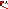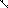# MINLPLib

### A Library of Mixed-Integer and Continuous Nonlinear Programming Instances

#### Instance st_robot

 Formatsⓘ ams gms lp mod nl osil pip py Primal Bounds (infeas ≤ 1e-08)ⓘ 0.00000000 p1 ( gdx sol ) (infeas: 5e-11) Other points (infeas > 1e-08)ⓘ Dual Boundsⓘ 0.00000000 (ANTIGONE)0.00000000 (BARON)0.00000000 (COUENNE)0.00000000 (GUROBI)0.00000000 (LINDO)0.00000000 (SCIP) Referencesⓘ Tawarmalani, M and Sahinidis, N V, Convexification and Global Optimization in Continuous and Mixed-Integer Nonlinear Programming: Theory, Algorithms, Software, and Applications, Kluwer, 2002.Tsai, L-W and Morgan, A P, Solving the kinematics of the most general six- and five-degree-of-freedom manipulators by continuation methods, Journal of Mechanics, Transmissions, and Automation in Design}", year = "1985, 107:2, 189-200. Sourceⓘ BARON book instance input/robot Added to libraryⓘ 03 Sep 2002 Problem typeⓘ QCP #Variablesⓘ 8 #Binary Variablesⓘ 0 #Integer Variablesⓘ 0 #Nonlinear Variablesⓘ 8 #Nonlinear Binary Variablesⓘ 0 #Nonlinear Integer Variablesⓘ 0 Objective Senseⓘ min Objective typeⓘ constant Objective curvatureⓘ linear #Nonzeros in Objectiveⓘ 0 #Nonlinear Nonzeros in Objectiveⓘ 0 #Constraintsⓘ 8 #Linear Constraintsⓘ 1 #Quadratic Constraintsⓘ 7 #Polynomial Constraintsⓘ 0 #Signomial Constraintsⓘ 0 #General Nonlinear Constraintsⓘ 0 Operands in Gen. Nonlin. Functionsⓘ Constraints curvatureⓘ indefinite #Nonzeros in Jacobianⓘ 24 #Nonlinear Nonzeros in Jacobianⓘ 16 #Nonzeros in (Upper-Left) Hessian of Lagrangianⓘ 14 #Nonzeros in Diagonal of Hessian of Lagrangianⓘ 8 #Blocks in Hessian of Lagrangianⓘ 5 Minimal blocksize in Hessian of Lagrangianⓘ 1 Maximal blocksize in Hessian of Lagrangianⓘ 3 Average blocksize in Hessian of Lagrangianⓘ 1.6 #Semicontinuitiesⓘ 0 #Nonlinear Semicontinuitiesⓘ 0 #SOS type 1ⓘ 0 #SOS type 2ⓘ 0 Minimal coefficientⓘ 1.6370e-03 Maximal coefficientⓘ 1.0000e+00 Infeasibility of initial pointⓘ 1 Sparsity JacobianⓘSparsity Hessian of Lagrangianⓘ```\$offlisting
*
*  Equation counts
*      Total        E        G        L        N        X        C        B
*          9        9        0        0        0        0        0        0
*
*  Variable counts
*                   x        b        i      s1s      s2s       sc       si
*      Total     cont   binary  integer     sos1     sos2    scont     sint
*          9        9        0        0        0        0        0        0
*  FX      0
*
*  Nonzero counts
*      Total    const       NL      DLL
*         25        9       16        0
*
*  Solve m using NLP minimizing objvar;

Variables  x1,x2,x3,x4,x5,x6,x7,x8,objvar;

Equations  e1,e2,e3,e4,e5,e6,e7,e8,e9;

e1.. 0.004731*x1*x3 - 0.1238*x1 - 0.3578*x2*x3 - 0.001637*x2 - 0.9338*x4 + x7
=E= 0.3571;

e2.. 0.2238*x1*x3 + 0.2638*x1 + 0.7623*x2*x3 - 0.07745*x2 - 0.6734*x4 - x7
=E= 0.6022;

e3.. x6*x8 + 0.3578*x1 + 0.004731*x2 =E= 0;

e4..  - 0.7623*x1 + 0.2238*x2 =E= -0.3461;

e5.. sqr(x1) + sqr(x2) =E= 1;

e6.. sqr(x3) + sqr(x4) =E= 1;

e7.. sqr(x5) + sqr(x6) =E= 1;

e8.. sqr(x7) + sqr(x8) =E= 1;

e9..    objvar =E= 0;

* set non-default bounds
x1.lo = -1; x1.up = 1;
x2.lo = -1; x2.up = 1;
x3.lo = -1; x3.up = 1;
x4.lo = -1; x4.up = 1;
x5.lo = -1; x5.up = 1;
x6.lo = -1; x6.up = 1;
x7.lo = -1; x7.up = 1;
x8.lo = -1; x8.up = 1;

Model m / all /;

m.limrow=0; m.limcol=0;
m.tolproj=0.0;

\$if NOT '%gams.u1%' == '' \$include '%gams.u1%'

\$if not set NLP \$set NLP NLP
Solve m using %NLP% minimizing objvar;

```

Last updated: 2023-08-16 Git hash: 2519540e